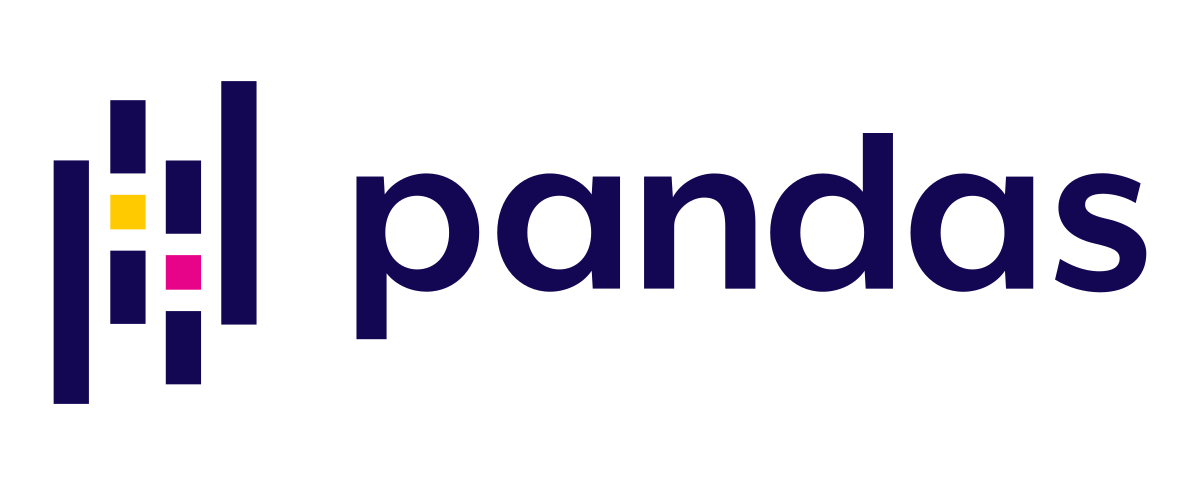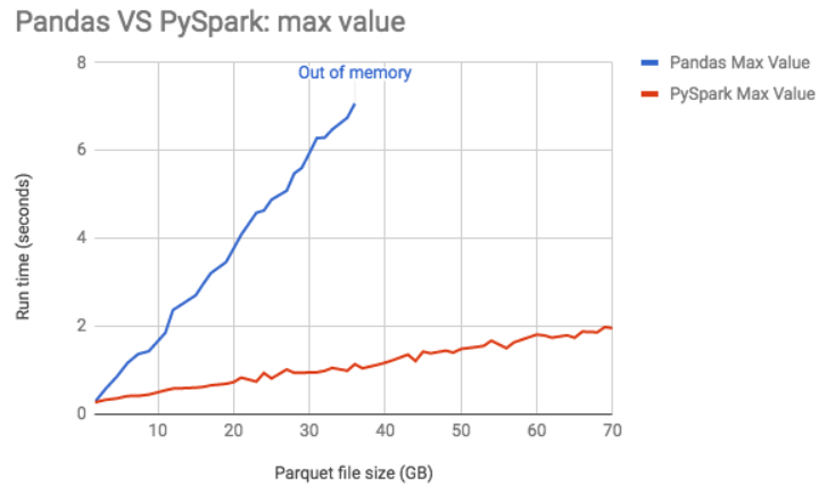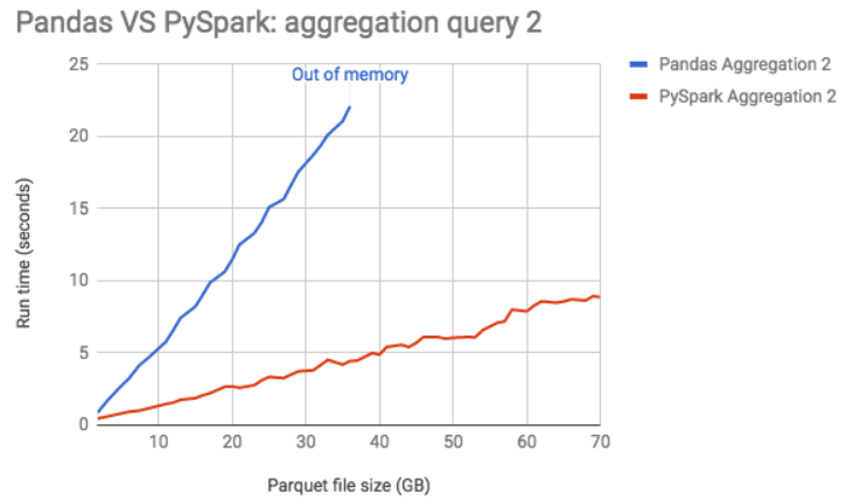# How to use Python and Pandas while embracing the power of Spark

If you have worked with data in Python before, you are probably familiar with the following line of code:

`import pandas as pd`The Pandas library is widely seen as the de-facto standard library for data science in Python and rightly so. It offers a fast, flexible and powerful tool to explore and manipulate data. Many data scientists rely daily on their Pandas-knowledge to analyze and tackle data-related use-cases.

While Pandas offers a great way to analyze data, Pandas has some shortcomings, mainly when working with big data. Pandas offers a great way to analyze data in Python, it requires the data to fit in-memory to do all the analytics and calculations. When working with big data, this might become tricky as the size of your data can easily surpass the amount of memory of your machine thus making queries slow & not scalable.

In this Insight I will explain how you can leverage the power of Spark in order to work with this big data still using your Pandas-knowledge.

### Working with big data

As mentioned before, working with big data is not straightforward in Pandas. All the data that you are working with, will be fully loaded in the memory of your machine when you are working with Pandas.

This means that you can only work with data that is smaller in size than the size of the memory of the machine you are working on. For example when you are working on a machine with 16 GB of RAM, you can only work with data that is smaller than 16 GB in size. Otherwise, an out of memory will be thrown.

In practice even, most of the times you can only work with data that is a fraction of your memory, because a part of your RAM is already occupied by other tasks. This limits you to work with big data in Pandas of course. The solution for this problem comes in the form of Spark.

### Enter 'Apache Spark'Apache Spark is a unified analytics/computing engine that enables distributed computing. What makes Spark great for our use-case when working with big data, is the fact that the data doesn’t need to fit in memory and data can be spilled to disk when it doesn’t fit into memory anymore. This allows us to work with data that is greater in size as the memory of the machine we are working on.

At its foundation, there is the Spark Core that takes care of distributed task dispatching, scheduling, and basic I/O functionalities. The Spark Core is exposed through APIs for Python, R, Java, Scala and .NET. On top of this core Spark offers four components/libraries (Spark SQL & Dataframes, Streaming, MLlib & GraphX).

These components offer great ways to e.g. work with batch and streaming data in a similar fashion (Spark Streaming) or to use scalable machine learning libraries (MLlib). For this insight however, the first component (Dataframes) will be the most important one. This allows us to work with structured data on Spark.

The Python API on Spark (PySpark) offers a way to work with these Dataframes in a Python way. However, there are quite some differences when you are used to Pandas; most importantly the syntax is truly different. This means that it comes with a learning curve.

### Differences between PySpark and Pandas

As mentioned before, (Py)Spark allows you to leverage the distributed power of Spark, which enables working with big data, compared to Pandas where we were limited to only work with data that fits in memory. This doesn’t only allow us to work with bigger data, but also to work faster with this (big) data as is seen in below graphs. These graphs show the run time for several queries in PySpark compared to the same queries in Pandas. It is clear that (Py)Spark indeed allows you to work with bigger data, in a more efficient way

#### Example PySpark vs. PandasEven wel calculating a simple max-value, Pandas can soon go out-of-memory when the dataset is too big

#### Example of a "COUNT DISTINCT" PySpark vs. Pandas?For some aggregations (e.g. count distincts) Pyspark also needs time but it still prevents out-of-memory issues in runtime

``SELECT count(distinct ss_customer_sk ) FROM store_sales``

#### Example of a "SUM" PySpark vs. Pandas?Often Pandas goes out-of-memory in aggregations like joins & unions. Pyspark can distribute these over multiple nodes and thus offer speed

``SELECT sum( ss_net_profit ) FROM store_sales GROUP BY ss_store_sk``

The main difference between working with PySpark and Pandas is the syntax. To show this difference, I provide a simple example of reading in a parquet file and doing some transformations on the data. As you can see, the syntax is completely different between PySpark and Pandas, which means that your Pandas knowledge is not directly transferable to PySpark.

``````# Pandas
pandasDF['SumOfTwoColumns'] = pandasDF['Column1'] + pandasDF['Column2']
pandasDF.rename({'Column1': 'Col1', 'Column2': 'Col2'}, axis=1, inplace=True)

# PySpark
sparkDF = sparkDF.withColumn('SumOfTwoColumns', col('Column1') + col('Column2'))
sparkDF = sparkDF.withColumnRenamed('Column1', 'Col1').withColumnRenamed('Column2', 'Col2')``````

These differences in usage, but also in syntax, mean that there is a learning curve when transferring from using pure Pandas code to pure PySpark code. This also means that your legacy Pandas code can not be used directly on Spark with PySpark. Luckily there are solutions that allow you to use your Pandas code and knowledge on Spark.

### Solutions to leverage the power of Spark with Pandas

There are mainly two options to use Pandas code on Spark: Koalas and Pandas UDFs#### Koalas

Koalas provides a Pandas dataframe API on Apache Spark. This means that – through koalas - you can use Pandas syntax on Spark dataframes. The main advantage with Koalas is that data scientists with Pandas knowledge can immediately be productive with Koalas on big data. On the other hand this allows you to have one code-base to work with smaller subsets of the data in Pandas and to work with big data in Spark (Koalas).

In the following example you can easily see that the syntax is exactly the same for Pandas and Koalas. The only difference is the second line of code, where respectively a pandas dataframe and a koalas dataframe is created. The operations that are done on both dataframes are exactly the same.

``````import pandas as pd

df = pd.DataFrame({"x": [1, 2], "y": [3, 4], "z": [5, 6]})
# Rename columns
df.columns = ["x", "y", "z1"]
# Do some operations in place
df["x2"] = df.x * df.x
``````
``````import databricks.koalas as ks

df = ks.DataFrame({"x": [1, 2], "y": [3, 4], "z": [5, 6]})
# Rename columns
df.columns = ["x", "y", "z1"]
# Do some operations in place
df["x2"] = df.x * df.x
``````

Koalas makes it easy to perform your well-known pandas operations on the power of Spark. However, because Koalas is still an ongoing project, not all Pandas functionalities are currently covered in Koalas. Around 80% of the Pandas functionalities are covered today.

To summarize Koalas I listed the Pro’s and Con’s:

Pro’s:

• Works with big data in Spark
• Re-uses your Pandas code and knowledge
• Perfects for EDA/Feature engineering you would normally do in Pandas

Con’s:

• Not all functionalities of Pandas are available (yet) in Koalas
• For other uses of Spark, you still would need to learn PySpark.
• Structured streaming, MLlib

#### Pandas UDFs

Pandas UDFs offer a second way to use Pandas code on Spark. With Pandas UDFs you actually apply a function that uses Pandas code on a Spark dataframe, which makes it a totally different way of using Pandas code in Spark.

User Defined Functions, or UDFs, allow you to define custom functions in Python and register them in Spark, this way you can execute these Python/Pandas functions on Spark dataframes.

There is an important difference between normal UDFs and Pandas UDFs. Normal UDFs get executed in a row-at-a-time way, which can make the execution of these UDFs on big dataframes very slow. Pandas UDFs, on the other hand, work as vectorized UDFs, which means that they are not executed row-at-a-time but in a vectorized way.

There are two types of Pandas UDFs: Scalar UDFs and Grouped Map UDFs. Scalar UDFs are used for vectorizing scalar operations while Grouped Map UDFs work in a split-apply-combine pattern.

You can easily define a function as a pandas UDF by adding ‘@(pandas_udf)’ above the function and defining the input and output type (for scalar UDFs). You are then able to use these Scalar UDFs on Spark dataframes in your queries, or use your Grouped Map UDFs with the applyInPandas() function, where you will also need to specify a schema of the dataframe that the UDF returns.

Example Scalar UDF:

``````import pandas as pd
from pyspark.sql.functions import pandas_udf

@pandas_udf('long')
def pandas_plus_one(s: pd.Series) -> pd.Series:
return s + 1

spark.range(10).select(pandas_plus_one("id")).show()``````

Example Grouped Map UDF:

``````import pandas as pd

df = spark.createDataFrame([(1, 1.0), (1, 2.0), (2, 3.0), (2, 5.0), (2, 10.0)], ("id", "v"))

def subtract_mean(pdf: pd.DataFrame) -> pd.DataFrame:
v = pdf.v
return pdf.assign(v=v - v.mean())

df.groupby("id").applyInPandas(subtract_mean, schema=df.schema).show()``````

### Example (very relevant) use-case: Parallelizing your Machine Learning with Grouped Map UDFs

A nice use case of Grouped Map UDFs occurs when you want to parallelize the training of machine learning algorithms with the split-apply-combine pattern that these UDFs offer.

With this, you can define a machine learning function (that works on a Pandas dataframe) that does your training and returns e.g. the predictions for a test set. You then group your Spark dataframe on a granularity that you want to train, e.g. a model for every customer-product combination (the split step) and afterwards you apply your defined training function to this grouped Spark dataframe object (the apply step). This will execute the function (your UDF) on these smaller dataframes (e.g. for every customer-product combination) and combines the results together in one Spark dataframe (combine step). This way you can easily create machine learning models for every customer-product combination in a distributed way.

``````def training_function(pandas_df):
# Your Training code on a pandas dataframe

results = ...
return results[["Date", "Customer", "Product", "Prediction"]]

return_schema = StructType([
StructField("Date", DateType()),
StructField("Customer", StringType()),
StructField("Product", StringType()),
StructField("Prediction", DoubleType())
])

results_df  = sales_df \
```            .groupBy("Customer", "Product")
.applyInPandas(training_function, schema = return_schema)```

### Conclusion

Koalas and Pandas UDFs offer two different ways to use your Pandas code and knowledge to leverage the power of Spark. Koalas offers all the ease, usability and syntax-knowledge of Pandas on Spark dataframes while Pandas UDFs give you the opportunity to apply custom functions with Pandas code on Spark dataframes. While you don't need anything other than your Pandas knowledge to use Koalas, Pandas UDFs still require you to learn PySpark syntax in order to be able to execute the UDFs. Either way these are both great solutions to work with big data while still using your Pandas knowledge.